Next: Motion with constant velocity Up: Motion in 1 dimension Previous: Velocity

## Acceleration

The conventional definition of acceleration is as follows:
Acceleration is the rate of change of velocity with time.
This definition implies that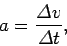(15)

whereis the body's acceleration at time, and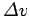is the change in velocity of the body between timesand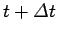.

How should we choose the time interval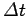appearing in Eq. (15)? Again, in the simple case in which the body is moving with constant acceleration, we can makeas large or small as we like, and it will not affect the value of. Suppose, however, thatis constantly changing in time, as is generally the case. In this situation,must be kept sufficiently small that the body's acceleration does not change appreciably between timesand.

A general expression for instantaneous acceleration, which is valid irrespective of how rapidly or slowly the body's acceleration changes in time, can be obtained by taking the limit of Eq. (15) asapproaches zero: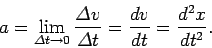(16)

The above definition is particularly useful if we can represent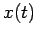as an analytic function, because it allows us to immediately evaluate the instantaneous acceleration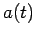via the rules of calculus. Thus, ifis given by formula (11) then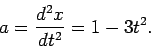(17)

Figure 5 shows the graph ofversus time obtained from the above expression. Note that whenis positive the body is accelerating to the right (i.e.,is increasing in time). Likewise, whenis negative the body is decelerating (i.e.,is decreasing in time).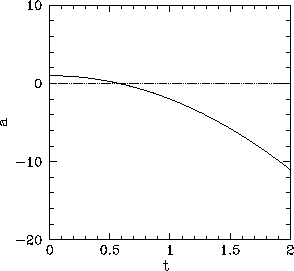Fortunately, it is generally not necessary to evaluate the rate of change of acceleration with time, since this quantity does not appear in Newton's laws of motion.Next: Motion with constant velocity Up: Motion in 1 dimension Previous: Velocity
Richard Fitzpatrick 2006-02-02